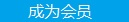您可以捐助，支持我们的公益事业。 1元 10元 50元 认证码：必填求知 文章 文库 Lib 视频 Code iProcess 课程 认证 咨询 工具 火云堂 讲座吧 成长之路要资料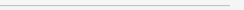订阅捐助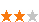382 次浏览     评价： 好 中 差
2018-10-26

 编辑推荐: 本文来自于csdn，本文中使用sklearn中的IRIS（鸢尾花）数据集来对特征处理功能进行说明。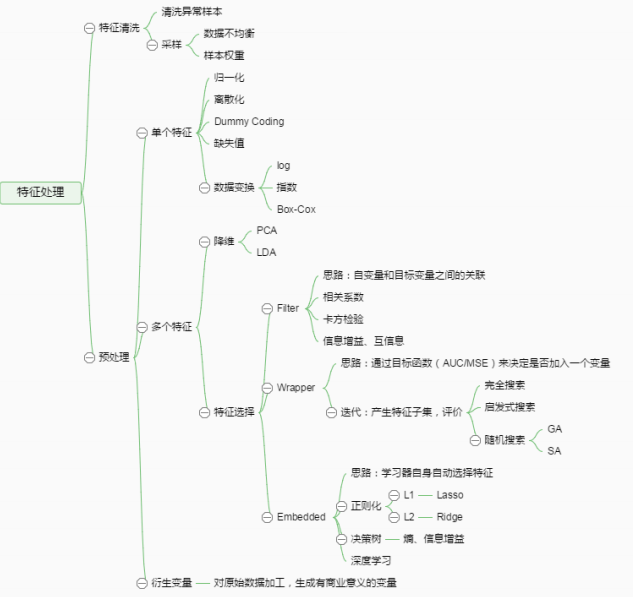from sklearn.datasets import load_iris #导入IRIS数据集 iris = load_iris() #特征矩阵 iris.data #目标向量 iris.target

2 数据预处理

1、不属于同一量纲：即特征的规格不一样，不能够放在一起比较。无量纲化可以解决这一问题。

2、信息冗余：对于某些定量特征，其包含的有效信息为区间划分，例如学习成绩，假若只关心“及格”或不“及格”，那么需要将定量的考分，转换成“1”和“0”表示及格和未及格。二值化可以解决这一问题。

3、定性特征不能直接使用：某些机器学习算法和模型只能接受定量特征的输入，那么需要将定性特征转换为定量特征。最简单的方式是为每一种定性值指定一个定量值，但是这种方式过于灵活，增加了调参的工作。通常使用哑编码的方式将定性特征转换为定量特征：假设有N种定性值，则将这一个特征扩展为N种特征，当原始特征值为第i种定性值时，第i个扩展特征赋值为1，其他扩展特征赋值为0。哑编码的方式相比直接指定的方式，不用增加调参的工作，对于线性模型来说，使用哑编码后的特征可达到非线性的效果。

4、存在缺失值：缺失值需要补充。

5、信息利用率低：不同的机器学习算法和模型对数据中信息的利用是不同的，之前提到在线性模型中，使用对定性特征哑编码可以达到非线性的效果。类似地，对定量变量多项式化，或者进行其他的转换，都能达到非线性的效果。

2.1 无量纲化

2.1.1 标准化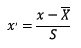from sklearn.preprocessing import StandardScaler #标准化，返回值为标准化后的数据 StandardScaler().fit_transform(iris.data)

2.1.2 区间缩放法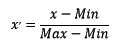from sklearn.preprocessing import MinMaxScaler #区间缩放，返回值为缩放到[0, 1]区间的数据 MinMaxScaler().fit_transform(iris.data)

2.1.3 标准化与归一化的区别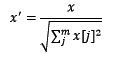from sklearn.preprocessing import Normalizer #归一化，返回值为归一化后的数据 Normalizer().fit_transform(iris.data)

2.2 对定量特征二值化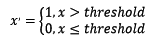from sklearn.preprocessing import Binarizer #二值化，阈值设置为3，返回值为二值化后的数据 Binarizer(threshold=3).fit_transform(iris.data)

2.3 对定性特征哑编码

 from sklearn.preprocessing import OneHotEncoder #哑编码，对IRIS数据集的目标值，返回值为哑编码 后的数OneHotEncoder().fit_transform(iris.target .reshape((-1,1)))

2.4 缺失值计算

 from numpy import vstack, array, nan from sklearn.preprocessing import Imputer #缺失值计算，返回值为计算缺失值后的数据 #参数missing_value为缺失值的表示形式，默认为NaN #参数strategy为缺失值填充方式，默认为mean（均值） Imputer().fit_transform(vstack((array([nan, nan, nan, nan]), iris.data)))

2.5 数据变换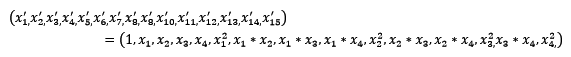from sklearn.preprocessing import PolynomialFeatures #多项式转换 #参数degree为度，默认值为2 PolynomialFeatures().fit_transform(iris.data)

 from numpy import log1p from sklearn.preprocessing import FunctionTransformer #自定义转换函数为对数函数的数据变换 #第一个参数是单变元函数 FunctionTransformer(log1p).fit_transform(iris.data)

2.6 回顾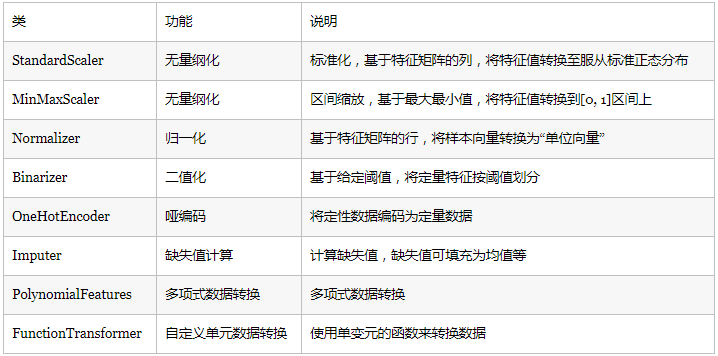3 特征选择

1、特征是否发散：如果一个特征不发散，例如方差接近于0，也就是说样本在这个特征上基本上没有差异，这个特征对于样本的区分并没有什么用。

2、特征与目标的相关性：这点比较显见，与目标相关性高的特征，应当优选选择。除方差法外，本文介绍的其他方法均从相关性考虑。

1、Filter：过滤法，按照发散性或者相关性对各个特征进行评分，设定阈值或者待选择阈值的个数，选择特征。

2、Wrapper：包装法，根据目标函数（通常是预测效果评分），每次选择若干特征，或者排除若干特征。

3、Embedded：嵌入法，先使用某些机器学习的算法和模型进行训练，得到各个特征的权值系数，根据系数从大到小选择特征。类似于Filter方法，但是是通过训练来确定特征的优劣。

3.1 Filter

3.1.1 方差选择法

 from sklearn.feature_selection import VarianceThreshold #方差选择法，返回值为特征选择后的数据 #参数threshold为方差的阈值 VarianceThreshold(threshold=3).fit_transform(iris.data)

3.1.2 相关系数法

 from sklearn.feature_selection import SelectKBest from scipy.stats import pearsonr #选择K个最好的特征，返回选择特征后的数据 #第一个参数为计算评估特征是否好的函数，该函数输入特征矩阵和目标向量，输出二元组（评分，P值）的数组，数组第i项为第i个特征的评分和P值。在此定义为计算相关系数 #参数k为选择的特征个数 SelectKBest(lambda X, Y: array(map(lambda x:pearsonr(x, Y), X.T)).T, k=2).fit_transform(iris.data, iris.target)

3.1.3 卡方检验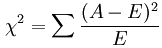from sklearn.feature_selection import SelectKBest from sklearn.feature_selection import chi2 #选择K个最好的特征，返回选择特征后的数据 SelectKBest(chi2, k=2).fit_transform(iris.data, iris.target)

3.1.4 互信息法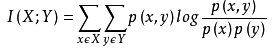from sklearn.feature_selection import SelectKBest from minepy import MINE #由于MINE的设计不是函数式的，定义mic方法将其为函数式的，返回一个二元组，二元组的第2项设置成固定的P值0.5 def mic(x, y): m = MINE() m.compute_score(x, y) return (m.mic(), 0.5) #选择K个最好的特征，返回特征选择后的数据 SelectKBest(lambda X, Y: array(map(lambda x:mic(x, Y), X.T)).T, k=2).fit_transform(iris.data, iris.target)

3.2.1 递归特征消除法

 from sklearn.feature_selection import RFE from sklearn.linear_model import LogisticRegression #递归特征消除法，返回特征选择后的数据 #参数estimator为基模型 #参数n_features_to_select为选择的特征个数 RFE(estimator=LogisticRegression(), n_features_to_select=2).fit_transform(iris.data, iris.target)

3.3 Embedded

3.3.1 基于惩罚项的特征选择法

 from sklearn.feature_selection import SelectFromModel from sklearn.linear_model import LogisticRegression #带L1惩罚项的逻辑回归作为基模型的特征选择 SelectFromModel(LogisticRegression(penalty="l1", C=0.1)).fit_transform(iris.data, iris.target)

L1惩罚项降维的原理在于保留多个对目标值具有同等相关性的特征中的一个，所以没选到的特征不代表不重要。故，可结合L2惩罚项来优化。具体操作为：若一个特征在L1中的权值为1，选择在L2中权值差别不大且在L1中权值为0的特征构成同类集合，将这一集合中的特征平分L1中的权值，故需要构建一个新的逻辑回归模型：

 from sklearn.linear_model import LogisticRegression class LR(LogisticRegression): def __init__(self, threshold=0.01, dual=False, tol=1e-4, C=1.0, fit_intercept=True, intercept_scaling=1, class_weight=None, random_state=None, solver='liblinear', max_iter=100, multi_class='ovr', verbose=0, warm_start=False, n_jobs=1): #权值相近的阈值 self.threshold = threshold LogisticRegression.__init__(self, penalty='l1', dual=dual, tol=tol, C=C, fit_intercept=fit_intercept, intercept_scaling=intercept_scaling, class_weight=class_weight, random_state=random_state, solver=solver, max_iter=max_iter, multi_class=multi_class, verbose=verbose, warm_start=warm_start, n_jobs=n_jobs) #使用同样的参数创建L2逻辑回归 self.l2 = LogisticRegression(penalty='l2', dual=dual, tol=tol, C=C, fit_intercept=fit_intercept, intercept_scaling=intercept_scaling, class_weight = class_weight, random_state=random_state, solver=solver, max_iter=max_iter, multi_class=multi_class, verbose=verbose, warm_start=warm_start, n_jobs=n_jobs) def fit(self, X, y, sample_weight=None): #训练L1逻辑回归 super(LR, self).fit(X, y, sample_weight=sample_weight) self.coef_old_ = self.coef_.copy() #训练L2逻辑回归 self.l2.fit(X, y, sample_weight=sample_weight) cntOfRow, cntOfCol = self.coef_.shape #权值系数矩阵的行数对应目标值的种类数目 for i in range(cntOfRow): for j in range(cntOfCol): coef = self.coef_[i][j] #L1逻辑回归的权值系数不为0 if coef != 0: idx = [j] #对应在L2逻辑回归中的权值系数 coef1 = self.l2.coef_[i][j] for k in range(cntOfCol): coef2 = self.l2.coef_[i][k] #在L2逻辑回归中，权值系数之差小于设定的阈值，且在L1中对应的权值为0 if abs(coef1-coef2) < self.threshold and j != k and self.coef_[i][k] == 0: idx.append(k) #计算这一类特征的权值系数均值 mean = coef / len(idx) self.coef_[i][idx] = mean return self

 from sklearn.feature_selection import SelectFromModel #带L1和L2惩罚项的逻辑回归作为基模型的特征选择 #参数threshold为权值系数之差的阈值 SelectFromModel(LR(threshold=0.5, C=0.1)).fit_transform(iris.data, iris.target)

3.3.2 基于树模型的特征选择法


from                                    sklearn.feature_selection                                    import SelectFromModel                                    from sklearn.ensemble import GradientBoostingClassifier                                                                       #GBDT作为基模型的特征选择                                    SelectFromModel(GradientBoostingClassifier()).                                 fit_transform(iris.data,                                    iris.target)

3.4 回顾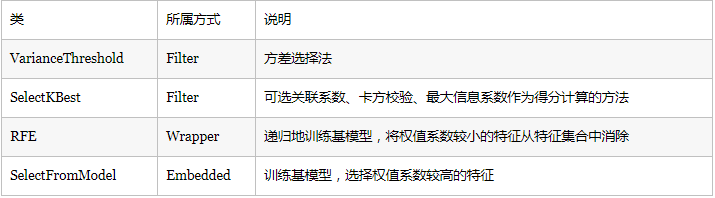4 降维

4.1 主成分分析法（PCA）

 from sklearn.decomposition import PCA #主成分分析法，返回降维后的数据 #参数n_components为主成分数目 PCA(n_components=2).fit_transform(iris.data)

4.2 线性判别分析法（LDA）

 from sklearn.lda import LDA #线性判别分析法，返回降维后的数据 #参数n_components为降维后的维数 LDA(n_components=2).fit_transform(iris.data, iris.target)

4.3 回顾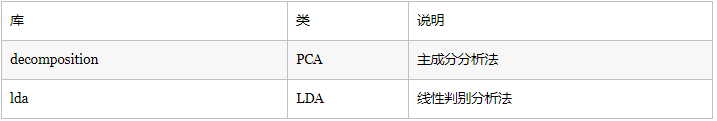382 次浏览  评价: 好 中 差订阅捐助
 相关文章 我们该如何设计数据库 数据库设计经验谈 数据库设计过程 数据库编程总结
 相关文档 数据库性能调优技巧 数据库性能调整 数据库性能优化讲座 数据库系统性能调优系列
 相关课程 高性能数据库设计与优化 高级数据库架构师 数据仓库和数据挖掘技术 Hadoop原理、部署与性能调优每天2个文档/视频 扫描微信二维码订阅
 订阅技术月刊 获得每月300个技术资源